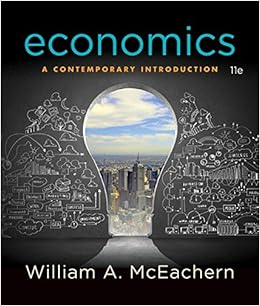# If the marginal propensity to consume is 75 and

• 15
• 100% (11) 11 out of 11 people found this document helpful

This preview shows page 12 - 15 out of 15 pages.

##### We have textbook solutions for you!
The document you are viewing contains questions related to this textbook.The document you are viewing contains questions related to this textbook.
Chapter 23 / Exercise 01
Economics: A Contemporary Introduction
McEachernExpert Verified
28. If the marginal propensity to consume is .75, and autonomous consumer spending increases by \$100 billion, the income expenditure model would predict that real GDP will increase by:A) \$100 billion.B) \$750 billion.C) \$400 billion.D) \$300 billion.
29. Suppose the marginal propensity to consume is equal to 0.90 and investment spending increases by \$50 billion. Assuming no taxes and no trade, by how much will real GDP change?
30. Suppose that a financial crisis decreases investment spending by \$100 billion and the marginal propensity to consume is 0.80. Assuming no taxes and no trade, by how much will real GDP change?
Page 12
##### We have textbook solutions for you!
The document you are viewing contains questions related to this textbook.The document you are viewing contains questions related to this textbook.
Chapter 23 / Exercise 01
Economics: A Contemporary Introduction
McEachernExpert Verified
Use the following to answer questions 31-32.Table: Disposable Income and Consumption31. (Table: Disposable Income and Consumption) Referring to the table provided, the autonomous consumer spending is:
32. (Table: Disposable Income and Consumption) Use the information in the table, the MPCis equal to:A) .80.B) 2.C) 1.20D) .60.
33. Consider a simple economy: MPC= 0.75, income =\$400 billion and aggregate consumption spending= \$400 billion. The autonomous consumption is:
Use the following to answer questions 34-38.Use this scenario to answer questions 155–163.Scenario: Income-Expenditure EquilibriumGDP is \$8,000, autonomous consumption is \$500, and planned investment spending is \$200. The marginal propensity to consume is 0.8. There are not taxes, transfers or government spending in this scenario.Page 13
34. (Scenario: Income-Expenditure Equilibrium) What is the consumption function?
YD
YD
YD
YD35. (Scenario: Income-Expenditure Equilibrium) How much is consumption?
36. (Scenario: Income-Expenditure Equilibrium) How much is total (planned) spending?A) \$7,100B) \$6,400C) \$8,000D) \$700
37. (Scenario: Income-Expenditure Equilibrium) How much is unplanned inventory investment?
38. (Scenario: Income-Expenditure Equilibrium) Given this situation, firms will tend to:
Page 14
Answer Key - Study Guide Midterm #2 B - 2.10
Page 15
•••